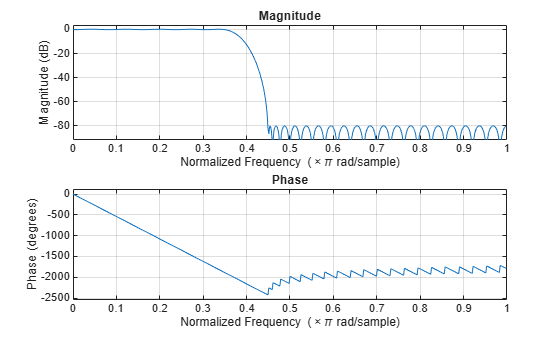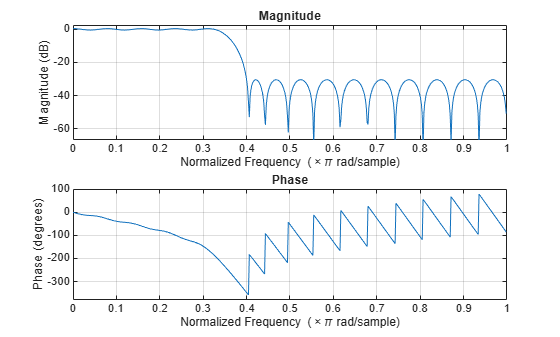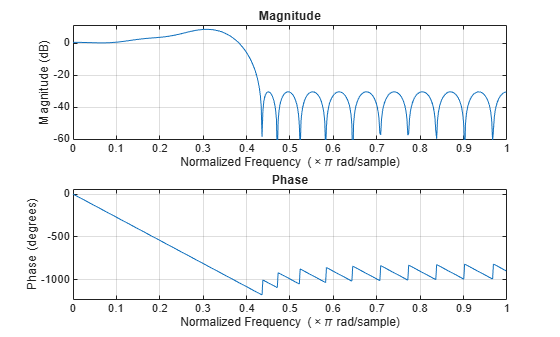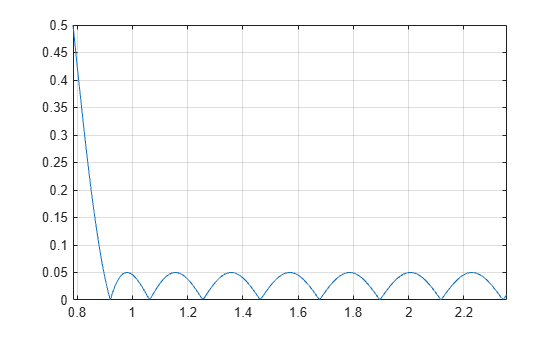Documentation

firceqrip

Constrained equiripple FIR filter

Syntax

B = firceqrip(n,Fo,DEV)
B = firceqrip(...,'slope',r)
B = firceqrip('minorder',[Fp Fst],DEV)
B = firceqrip(...,'passedge')
B = firceqrip(...,'stopedge')
B = firceqrip(...,'high')
B = firceqrip(...,'min')
B = firceqrip(...,'invsinc',C)
B = firceqrip(...,'invdiric',C)

Description

B = firceqrip(n,Fo,DEV) designs an order n filter (filter length equal n + 1) lowpass FIR filter with linear phase.

firceqrip produces the same equiripple lowpass filters that firpm produces using the Parks-McClellan algorithm. The difference is how you specify the filter characteristics for the function.

The input argument Fo specifies the frequency at the upper edge of the passband in normalized frequency (0<Fo<1). The two-element vector dev specifies the peak or maximum error allowed in the passband and stopbands. Enter [d1 d2] for dev where d1 sets the passband error and d2 sets the stopband error.

B = firceqrip(...,'slope',r) uses the input keyword 'slope' and input argument r to design a filter with a nonequiripple stopband. r is specified as a positive constant and determines the slope of the stopband attenuation in dB/normalized frequency. Greater values of r result in increased stopband attenuation in dB/normalized frequency.

B = firceqrip('minorder',[Fp Fst],DEV) designs filter with the minimum number of coefficients required to meet the deviations in DEV = [d1 d2] while having a transition width no greater than FstFp, the difference between the stopband and passband edge frequencies. You can specify 'mineven' or 'minodd' instead of 'minorder' to design minimum even order (odd length) or minimum odd order (even length) filters, respectively. The 'minorder' option does not apply when you specify the 'min' (minimum-phase), 'invsinc', or the 'invdiric' options.

B = firceqrip(...,'passedge') designs a filter where Fo specifies the frequency at which the passband starts to rolloff.

B = firceqrip(...,'stopedge') designs a filter where Fo specifies the frequency at which the stopband begins.

B = firceqrip(...,'high') designs a high pass FIR filter instead of a lowpass filter.

B = firceqrip(...,'min') designs a minimum-phase filter.

B = firceqrip(...,'invsinc',C) designs a lowpass filter whose magnitude response has the shape of an inverse sinc function. This may be used to compensate for sinc-like responses in the frequency domain such as the effect of the zero-order hold in a D/A converter. The amount of compensation in the passband is controlled by C, which is specified as a scalar or two-element vector. The elements of C are specified as follows:

• If C is supplied as a real-valued scalar or the first element of a two-element vector, firceqrip constructs a filter with a magnitude response of 1/sinc(C*pi*F) where F is the normalized frequency.

• If C is supplied as a two-element vector, the inverse-sinc shaped magnitude response is raised to the positive power C(2). If we set P=C(2), firceqrip constructs a filter with a magnitude response 1/sinc(C*pi*F)P.

If this FIR filter is used with a cascaded integrator-comb (CIC) filter, setting C(2) equal to the number of stages compensates for the multiplicative effect of the successive sinc-like responses of the CIC filters.

Note

Since the value of the inverse sinc function becomes unbounded at C=1/F, the value of C should be greater the reciprocal of the passband edge frequency. This can be expressed as Fo<1/C. For users familiar with CIC decimators, C is equal to 1/2 the product of the differential delay and decimation factor.

B = firceqrip(...,'invdiric',C) designs a lowpass filter with a passband that has the shape of an inverse Dirichlet sinc function. The frequency response of the inverse Dirichlet sinc function is given bywhere C, r, and p are scalars. The input C can be a scalar or vector containing 2 or 3 elements. If C is a scalar, p and r equal 1. If C is a two-element vector, the first element is C and the second element is p, [C p]. If C is a three-element vector, the third element is r, [C p r].

Examples

To introduce a few of the variations on FIR filters that you design with firceqrip, these five examples cover both the default syntax b = firceqrip(n,wo,del) and some of the optional input arguments. For each example, the input arguments n, wo, and del remain the same.

Filter design using firceqrip

Design a 30th order FIR filter using firceqrip.

b = firceqrip(30,0.4,[0.05 0.03]); fvtool(b)Design a minimum order FIR filter using firceqrip. The passband edge and stopband edge frequencies are 0.35$\pi$ and 0.45$\pi$ rad/sample. The allowed deviations are 0.02 and 1e-4.

b = firceqrip('minorder',[0.35 0.45],[0.02 1e-4]); fvtool(b)Design a 30th order FIR filter with the stopedge keyword to define the response at the edge of the filter stopband.

b = firceqrip(30,0.4,[0.05 0.03],'stopedge'); fvtool(b)Design a 30th order FIR filter with the slope keyword and r = 20.

b = firceqrip(30,0.4,[0.05 0.03],'slope',20,'stopedge'); fvtool(b)Design a 30th order FIR filter defining the stopband and specifying that the resulting filter is minimum phase with the min keyword.

b = firceqrip(30,0.4,[0.05 0.03],'stopedge','min'); fvtool(b)Comparing this filter to the filter in Figure 1. The cutoff frequency wo = 0.4 now applies to the edge of the stopband rather than the point at which the frequency response magnitude is 0.5.

Viewing the zero-pole plot shown here reveals this is a minimum phase FIR filter - the zeros lie on or inside the unit circle, z = 1

fvtool(b,'polezero')Design a 30th order FIR filter with the invsinc keyword to shape the filter passband with an inverse sinc function.

b = firceqrip(30,0.4,[0.05 0.03],'invsinc',[2 1.5]); fvtool(b)The inverse sinc function being applied is defined as 1/sinc(2*w)^1.5.

Inverse-Dirichlet-Sinc-Shaped Passband

Design two order 30 constrained equiripple FIR filters with inverse-Dirichlet-sinc-shaped passbands. The cutoff frequency in both designs is pi/4 radians/sample. Set C=1 in one design C=2 in the second design. The maximum passband and stopband ripple is 0.05. Set p=1 in one design and p=2 in the second design.

Design the filters.

b1 = firceqrip(30,0.25,[0.05 0.05],'invdiric',[1 1]);
b2 = firceqrip(30,0.25,[0.05 0.05],'invdiric',[2 2]);

Obtain the filter frequency responses using freqz. Plot the magnitude responses.

[h1,~] = freqz(b1,1);
[h2,w] = freqz(b2,1);
plot(w,abs(h1)); hold on;
plot(w,abs(h2),'r');
axis([0 pi 0 1.5]);
ylabel('Magnitude');
legend('C=1 p=1','C=2 p=2');Inspect the stopband ripple in the design with C=1 and p=1. The constrained design sets the maximum ripple to be 0.05. Zoom in on the stopband from the cutoff frequency of pi/4 radians/sample to 3pi/4 radians/sample.

figure;
plot(w,abs(h1));
set(gca,'xlim',[pi/4 3*pi/4]);
grid on;